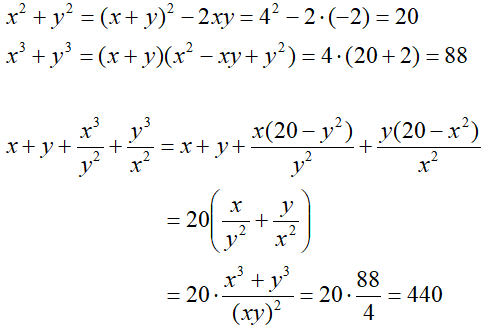# 請教一題求解

x+y=4
xy=-2

TOP

## 回覆 1# dtc5527 的帖子TOP

## 回覆 1# dtc5527 的帖子

x^2 + y^2 = (x + y)^2 - 2xy = 20

x^3 + y^3 = (x + y)^3 - 3xy(x + y) = 88

x + x^3/y^2 + y^3/x^2 + y
= (x^3y^2 + x^5 + y^5 + x^2y^3) / (x^2y^2)
= (x^2 + y^2)(x^3 + y^3) / (xy)^2
= 20 * 88 / 4
= 440

TOP

TOP

TOP

﻿